# Map scale Ratio of the distance on the

• Slides: 20Map scale � � � Ratio of the distance on the map to the distance on the ground Scale is a fraction Larger area covered means larger denominator Larger denominator means smaller fraction So a large-scale map covers a small areaLarge-scale Small-scale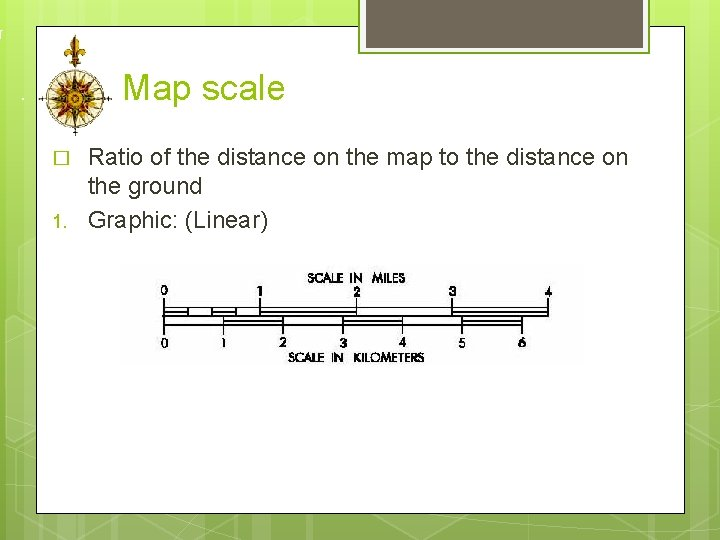Map scale � 1. Ratio of the distance on the map to the distance on the ground Graphic: (Linear)Map scale 2. Verbal: 1 cm represents 100, 000 � Easy to understand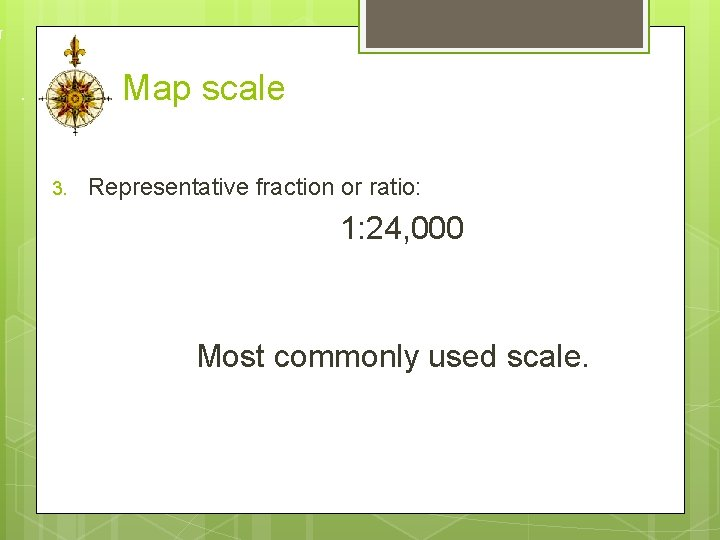Map scale 3. Representative fraction or ratio: 1: 24, 000 Most commonly used scale.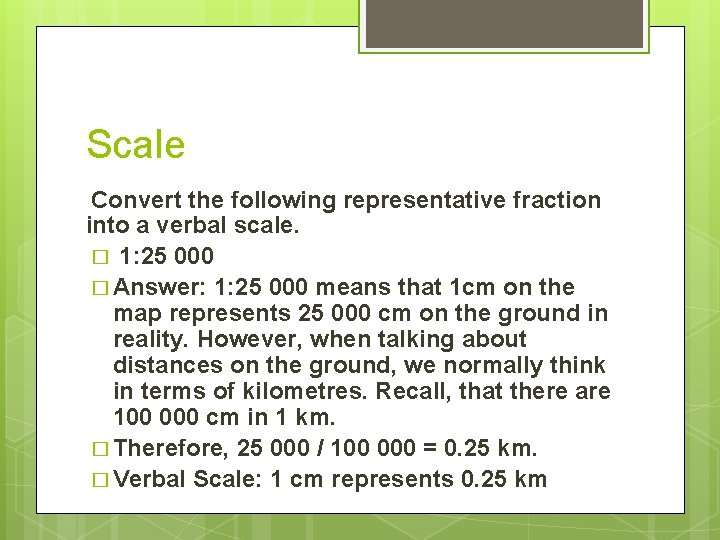Scale Convert the following representative fraction into a verbal scale. � 1: 25 000 � Answer: 1: 25 000 means that 1 cm on the map represents 25 000 cm on the ground in reality. However, when talking about distances on the ground, we normally think in terms of kilometres. Recall, that there are 100 000 cm in 1 km. � Therefore, 25 000 / 100 000 = 0. 25 km. � Verbal Scale: 1 cm represents 0. 25 km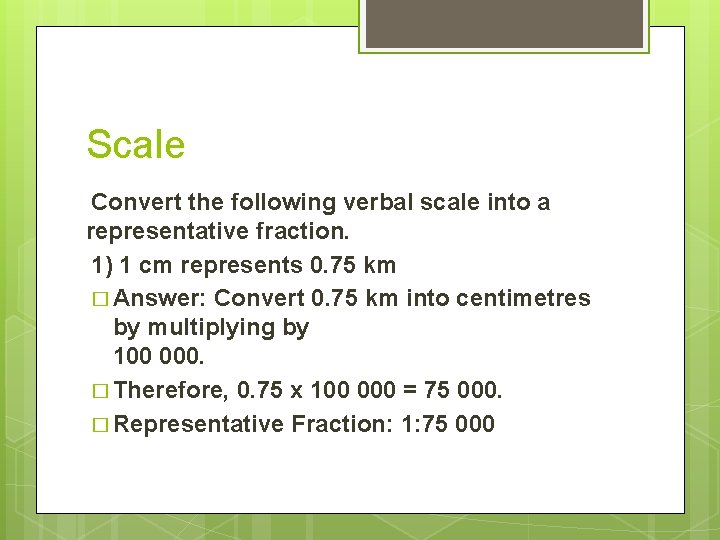Scale Convert the following verbal scale into a representative fraction. 1) 1 cm represents 0. 75 km � Answer: Convert 0. 75 km into centimetres by multiplying by 100 000. � Therefore, 0. 75 x 100 000 = 75 000. � Representative Fraction: 1: 75 000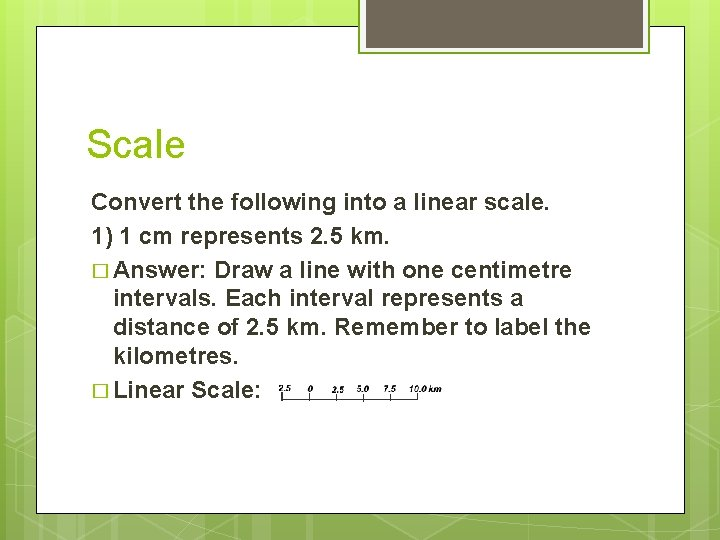Scale Convert the following into a linear scale. 1) 1 cm represents 2. 5 km. � Answer: Draw a line with one centimetre intervals. Each interval represents a distance of 2. 5 km. Remember to label the kilometres. � Linear Scale:Scale � Find the actual distance along the road from Town A to Town B on the map below. � Answer: Measure the first segment along the road from A and then the remaining segment to B. Note: Measure to one decimal place accuracy.Scale � Therefore, the total map distance along the road from A to B is 5. 8 cm. � Now, apply the scale. 1: 60 000 means that every centimetre on the map represents 0. 6 km in reality. � the actual distance between Town A and Town B is: 5. 8 cm x 0. 6 km = 3. 48 km.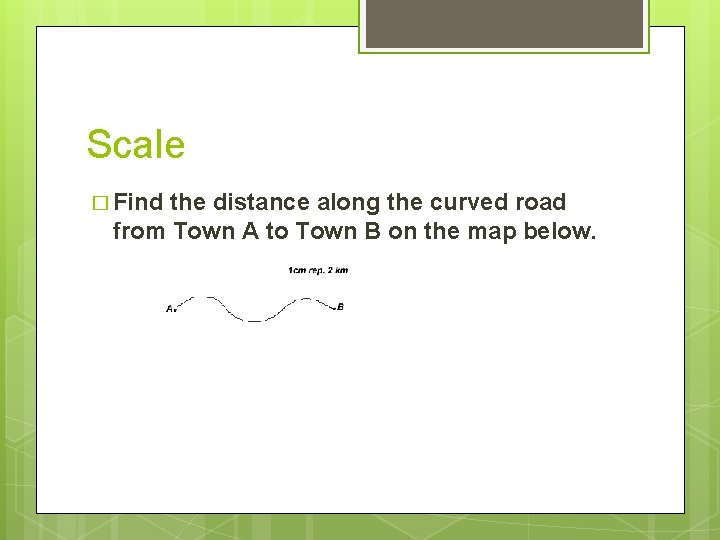Scale � Find the distance along the curved road from Town A to Town B on the map below.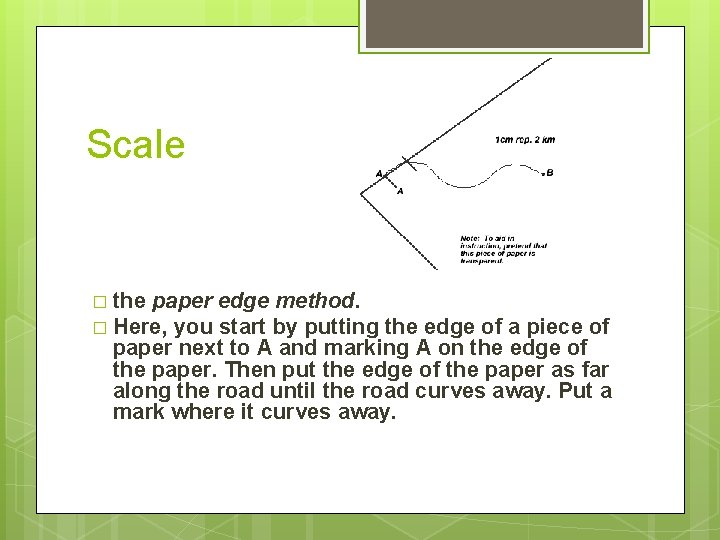Scale � the paper edge method. � Here, you start by putting the edge of a piece of paper next to A and marking A on the edge of the paper. Then put the edge of the paper as far along the road until the road curves away. Put a mark where it curves away.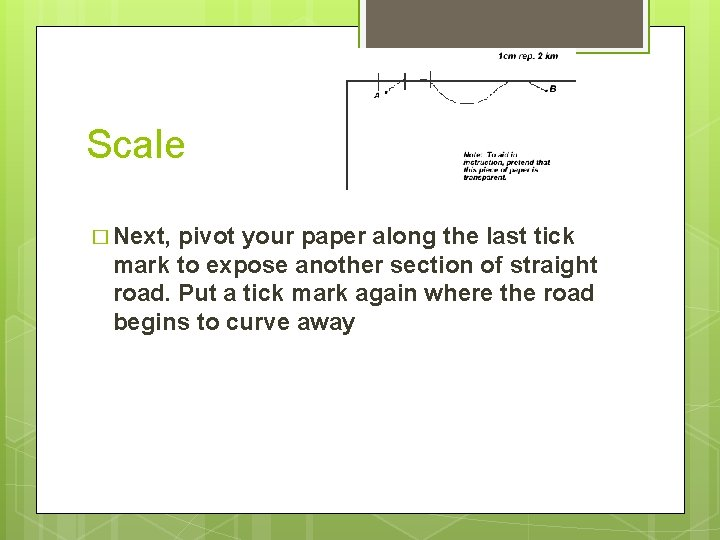Scale � Next, pivot your paper along the last tick mark to expose another section of straight road. Put a tick mark again where the road begins to curve awayScale � Continue in a similar fashion until you reach B. Then take your ruler and measure the distance between A and B on your paper to one decimal place accuracy. In this example, the distance is approximately 6. 4 cm. Then apply the scale. � 6. 4 cm x 2 km = 12. 8 km which is the actual distance between Town A and Town B.Scale � Scale Exercises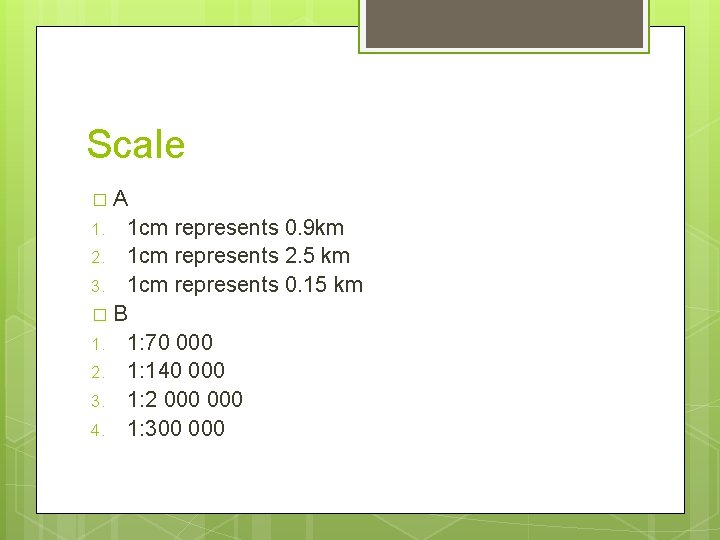Scale �A 1 cm represents 0. 9 km 2. 1 cm represents 2. 5 km 3. 1 cm represents 0. 15 km �B 1. 1: 70 000 2. 1: 140 000 3. 1: 2 000 4. 1: 300 000 1.Scale �D � EF = 2. 8 cm (acceptable range 2. 7 cm to 2. 9 CM 0 � Actual Distance 2. 8 cm X 2. 5 km (scale) = 7. o km � EF = 4. 5 cm � AD 4. 5 cm X 2. 5 km = 11. 25 km or 11. 3 km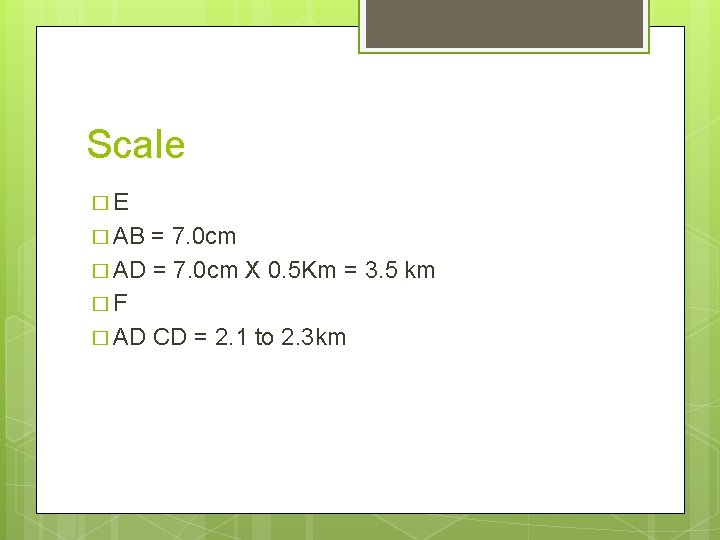Scale �E � AB = 7. 0 cm � AD = 7. 0 cm X 0. 5 Km = 3. 5 km �F � AD CD = 2. 1 to 2. 3 kmScale